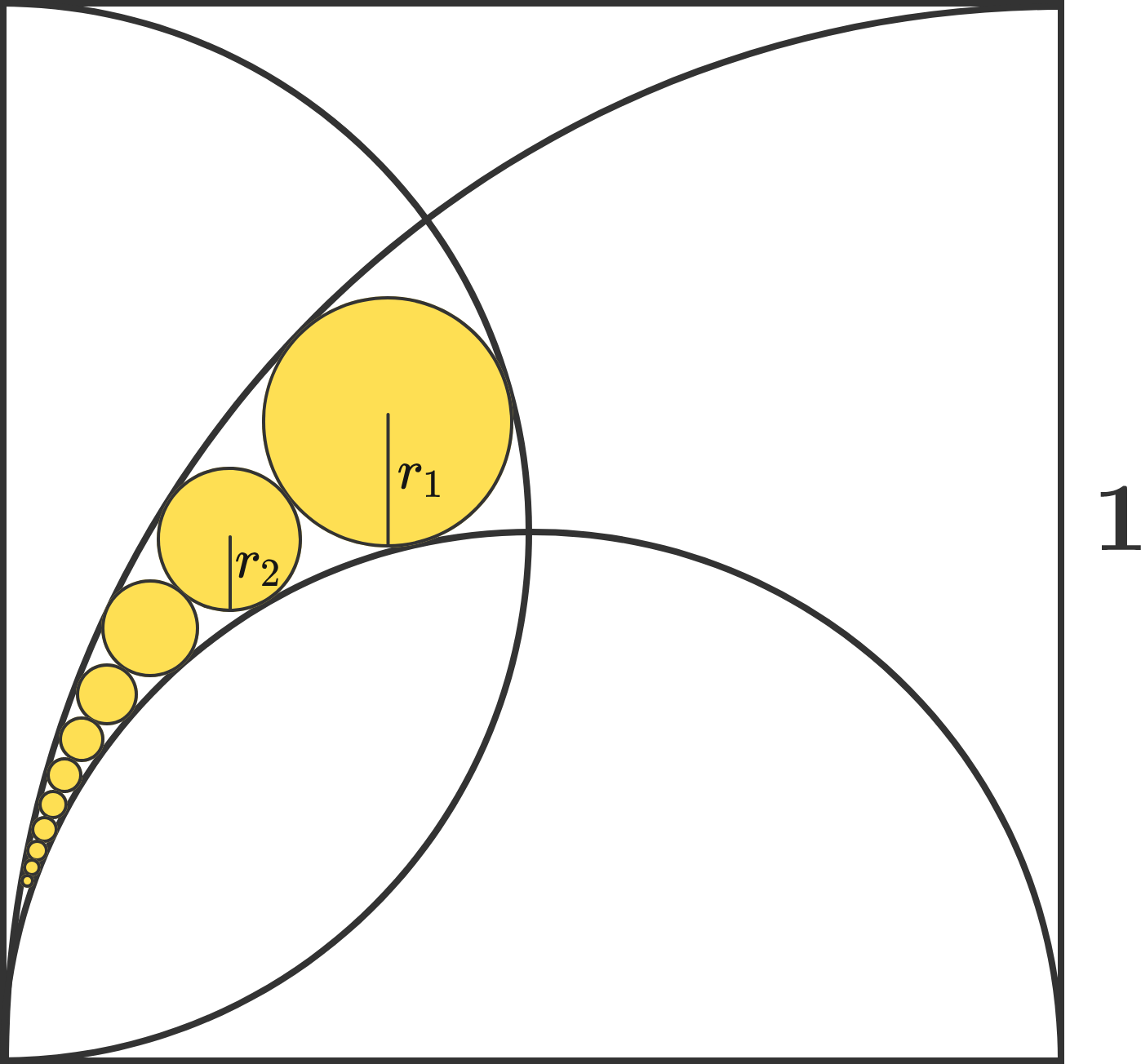# Two semicircles and a quarter of circle are inscribed in the square.What is the correct alternative?

Geometry Level 1

In the diagram below, two semicircles and one quarter-circle are inscribed in a unit square.

Inside one of the partitioned sections, infinitely many circles are drawn that are tangent to each other and to the boundaries. Let $r_n$ denote the radius of the $n^\text{th}$ largest circle.

Express $r_n$ in terms of $n.$×